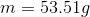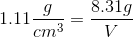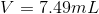# Homework 70

$$\newcommand{\vecs}{\overset { \rightharpoonup} {\mathbf{#1}} }$$ $$\newcommand{\vecd}{\overset{-\!-\!\rightharpoonup}{\vphantom{a}\smash {#1}}}$$$$\newcommand{\id}{\mathrm{id}}$$ $$\newcommand{\Span}{\mathrm{span}}$$ $$\newcommand{\kernel}{\mathrm{null}\,}$$ $$\newcommand{\range}{\mathrm{range}\,}$$ $$\newcommand{\RealPart}{\mathrm{Re}}$$ $$\newcommand{\ImaginaryPart}{\mathrm{Im}}$$ $$\newcommand{\Argument}{\mathrm{Arg}}$$ $$\newcommand{\norm}{\| #1 \|}$$ $$\newcommand{\inner}{\langle #1, #2 \rangle}$$ $$\newcommand{\Span}{\mathrm{span}}$$ $$\newcommand{\id}{\mathrm{id}}$$ $$\newcommand{\Span}{\mathrm{span}}$$ $$\newcommand{\kernel}{\mathrm{null}\,}$$ $$\newcommand{\range}{\mathrm{range}\,}$$ $$\newcommand{\RealPart}{\mathrm{Re}}$$ $$\newcommand{\ImaginaryPart}{\mathrm{Im}}$$ $$\newcommand{\Argument}{\mathrm{Arg}}$$ $$\newcommand{\norm}{\| #1 \|}$$ $$\newcommand{\inner}{\langle #1, #2 \rangle}$$ $$\newcommand{\Span}{\mathrm{span}}$$

## Q2.24

A.Sodium Hypochlorite (bleach) has a density of 1.11 g/cm3 and a volume of 48.21 mL, what is the mass?

B. What is the volume of 8.31 g of bleach?

Solution

a. m= 53.51g

b. V= 7.49mL

Strategy for part A

1. Recognize the equation for density is density (d) equals mass (m) divided by volume (V)2. Plug in the provided information from the question above3. Now convert cm3 into mL using the conversion factor 1 cm3=1 mL, these units should then cancel each other out leaving only grams as the unit. (This is what mass is measured in)

4.Now solve for m (mass).Strategy for part B

1. Use the density formula from part a to plug in the information given in the question above2. Plug in the values from the question above3. To get volume in mL, convert cm3 to mL use the conversion factor 1 cm3= 1 mL (This is what volume is measured in)

4. Solve for V## Q3.57

If an electron moves from a 3p orbital to a 2p orbital, does it give off energy or gain energy? Why?

Strategy

1. Identify the location of each orbital and how this relates to their energy levels

2. Explain why your answer is correct, referring to the movement of photons